# Samacheer Kalvi 12th Maths Guide Chapter 11 Probability Distributions Ex 11.3

Tamilnadu State Board New Syllabus Samacheer Kalvi 12th Maths Guide Pdf Chapter 11 Probability Distributions Ex 11.3 Textbook Questions and Answers, Notes.

## Tamilnadu Samacheer Kalvi 12th Maths Solutions Chapter 11 Probability Distributions Ex 11.3

Question 1.
The probability density function of X is given by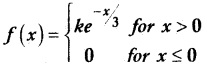Find the value of k.
Solution:
Since f(x) is a probability density function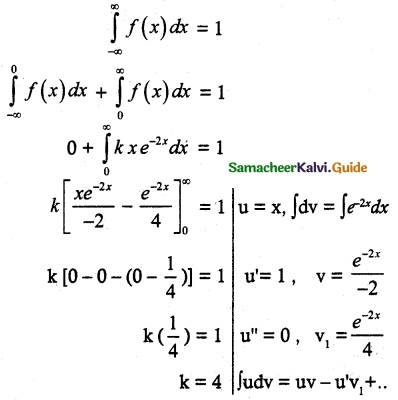Question 2.
The probability density function of X isFind
(i) P(0.2 ≤ X < 0.6)
(ii) P(1.2 ≤ X < 1.8)
(iii) P(0.5 ≤ X < 1.5)
Solution: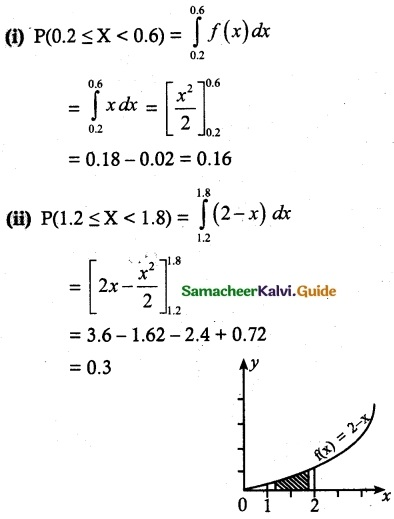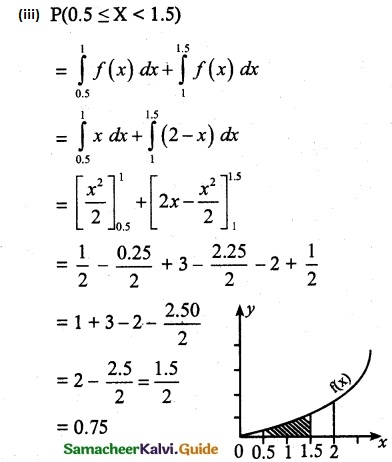Question 3.
Suppose the amount of milk sold daily at a milk booth is distributed with a minimum of 200 litres and a maximum of 600 litres with probability density functionFind
(i) the value of k
(ii) the distribution function
(iii) the probability that daily sales will fall between 300 litres and 500 litres?
Solution:(i) Since f(x) is a probability density function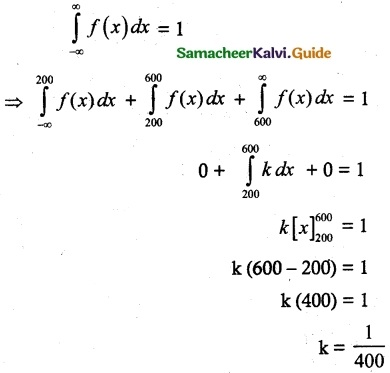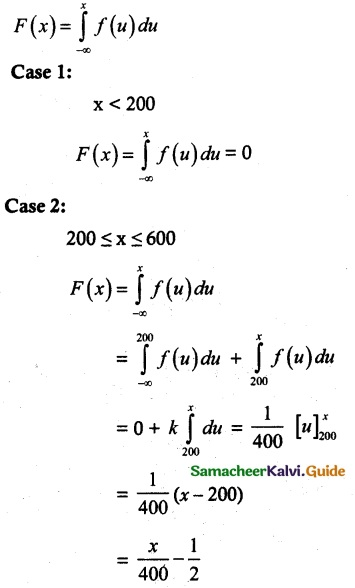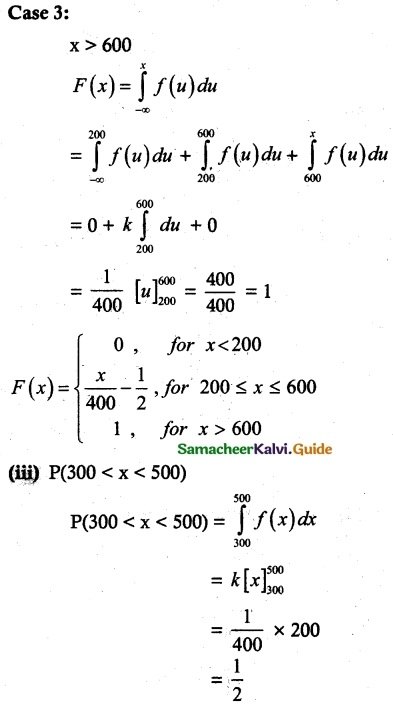Question 4.
The probability density function of X is given byFind
(i) the value of k
(ii) the distribution function
(iii) P(X < 3)
(iv) P(5 ≤ X)
(v) P(X ≤ 4)
Solution:
(i) Since f is a probability density function(ii) The distribution function F(x) = $$\int_{-\infty}^{x}$$ f(u) du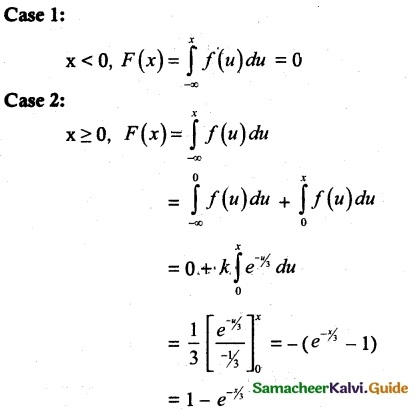Question 5.
If X is the random variable with probability density function f(x) given by,then find
(i) the distribution function F(x)
(ii) P(-0.5 ≤ x ≤ 0.5)
Solution:
Distribution function F(x) = $$\int_{-\infty}^{x}$$ f(u) du
case 1.
x < -1
F(x) = $$\int_{-\infty}^{x}$$ f(u) du = 0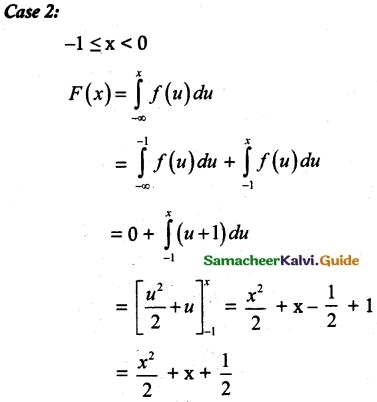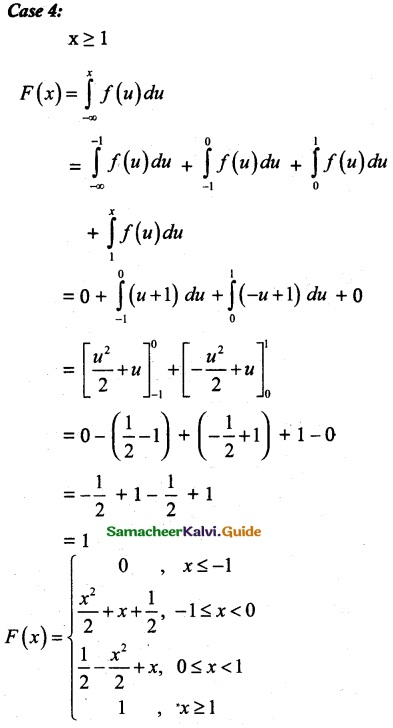Question 6.
If X is the random variable with distribution function F(x) given by,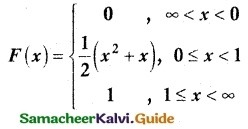Find
(i) the probability density function f(x)
(ii) P(0.3 ≤ X ≤ 0.6)
Solution:
(i) Probability density function(ii) P(0.3 ≤ X ≤ 0.6)
P(a ≤ X ≤ b) = F(b) – F(a)
P(0.3 ≤ X ≤ 0.6) = F(0.6) – F(0.3)
= $$\frac { 1 }{ 2 }$$ [(0.6)² + (0.6)] – $$\frac { 1 }{ 2 }$$ [(0.3)² + (0.3)]
= $$\frac { 1 }{ 2 }$$ (0.96 – 0.39)
= 0.285Scroll to Top